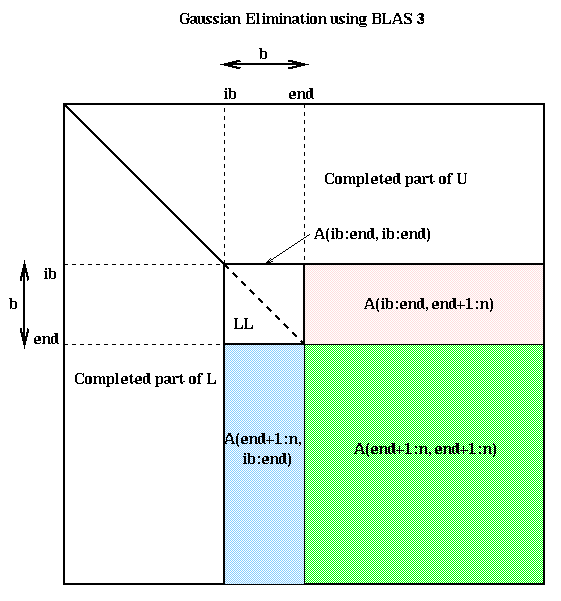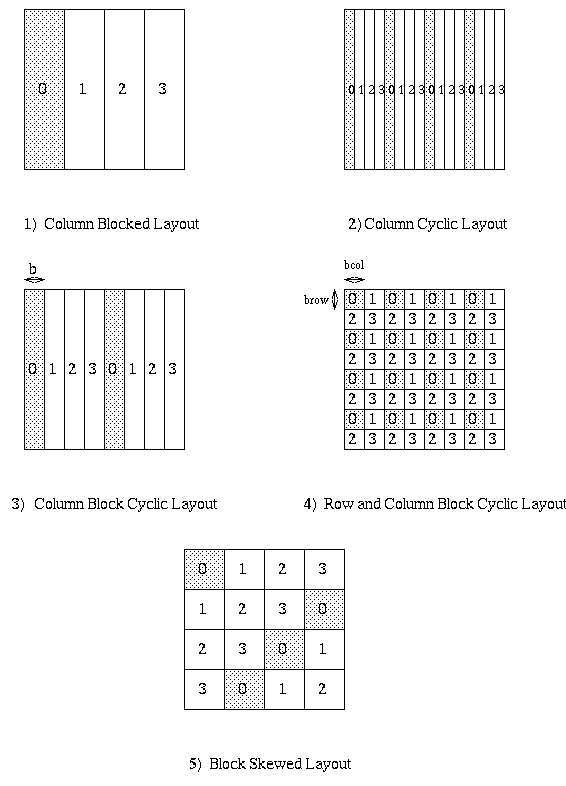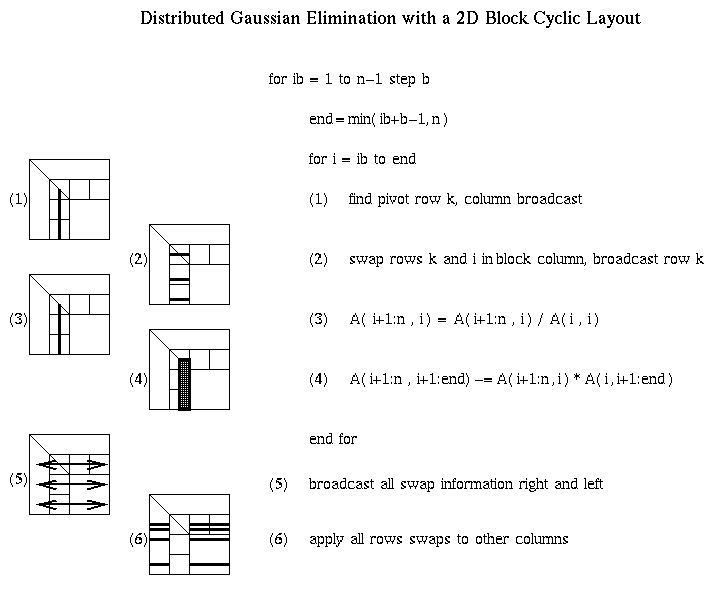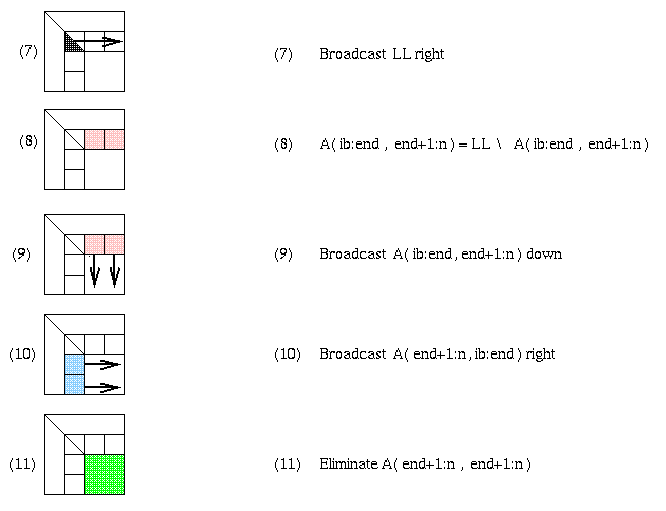# (CS 267, Feb 28 1995)

## How to Layout Matrices on Distributed Memory Machines

Now we consider data layout of matrices on distributed memory machines, with the goal of making Gaussian elimination as efficient as possible. We will discuss a sequence of data layouts, starting with the most simple, obvious, and inefficient one, and work our way up to the more complicated but efficient one ultimately used. Even though our justification is based on Gaussian elimination, analysis of many other algorithms has led to the same set of layouts. As a result, these layouts have been standardized as part of the High Performance Fortran standard, with corresponding data declarations as part of that language.

The two main issues in choosing a data layout for Gaussian elimination are

• load balance, or splitting the work reasonably evenly among the processors throughout the algorithm, and
• ability to use the BLAS3 during computations on a single processor, to account for the memory hierarchy on each processor.
• It will help to remember the pictorial representation of Gaussian elimination below. As the algorithm proceeds, it works on successively smaller square southeast corners of the matrix. In addition, there is extra BLAS2 work to factorize the submatrix A(ib:n,ib:end).For convenience we will number the processors from 0 to p-1, and matrix columns (or rows) from 0 to n-1. The following figure shows a sequence of data layouts we will consider. In all cases, each submatrix is labeled with the number of the processor (from 0 to 3) that contains it. Processor 0 owns the shaded submatrices.Consider the first layout, the Column Blocked Layout. In this layout, column i is stored on processor floor(i/c) where c=ceiling(n/p) is the maximum number of columns stored per processor. In the figure n=16 and p=4. This layout does not permit good load balancing, because as soon as the first c columns are complete, processor 0 is idle for the rest of the computation. The transpose of the this layout, the Row Blocked Layout, has a similar problem.

The second layout, the Column Cyclic Layout, tried to address this problem by assigning column i to processor i mod p. In the figure, n=16 and p=4. With this layout, each processor owns approximately 1/p-th of the square southeast corner of the matrix, so the load balance is good. However, the fact that single columns are stored rather than blocks means we cannot use the BLAS2 to factorize A(ib:n,ib:end), and may not be able to use the BLAS3 to update A(end+1:n,end+1:n). The transpose of this layout, the Row Cyclic Layout, has a similar problem.

The third layout, the Column Block Cyclic Layout, is a compromise between the last two. We choose a block size b, divide the columns into groups of size b, and distribute these groups in a cyclic manner. This means column i is stored in processor (floor(i/b)) mod p. In fact, this layout includes the first two as the special cases b=c=ceiling(n/p) and b=1, respectively. In the figure n=16, p=4 and b=2. For b larger than 1, this has a slightly worse balance than the Column Cyclic Layout, but can use the BLAS2 and BLAS3. For b less than c, it has a better load balance than the Columns Blocked Layout, but can only call the BLAS on smaller subproblems, and so perhaps take less advantage of the local memory hierarchy (recall our plot of performance of the BLAS3, where performance improved for larger matrix sizes). However, this layout has the disadvantage that the factorization of A(ib:n,ib:end) will take place on perhaps just on processor (in the natural situation where column blocks in the layout correspond to column blocks in Gaussian elimination). This would be a serial bottleneck.

This serial bottleneck is eased by the fourth layout in the figure, the Row and Column (or 2D) Block Cyclic Layout. Here we think of our p processors arranged in an prow-by-pcol rectangular array of processors, indexed in a 2D fashion by (pi,pj), 0 <= pi < prow and 0 <= pj < pcol. All the processors (i,j) with a fixed j are referred to as processor column j. All the processors (i,j) with a fixed i are referred to as processor row i. Matrix entry (i,j) is mapped to processor (pi,pj) by mapping i to pi and j to pj independently using the formula for a block cyclic layout:

```    pi = floor(i/brow) mod prow, where brow = block size in the row direction
pj = floor(j/bcol) mod pcol, where bcol = block size in the column direction
```
Thus, this layout includes all the previous layouts, and their transposes, as special cases. In the figure, n=16, p=4, prow=pcol=2, and brow=bcol=2. This layout permits pcol-fold parallelism in any column, and calls to the BLAS2 and BLAS3 on matrices of size brow-by-bcol. This is the layout that we will use for Gaussian elimination.

Since Gaussian elimination is not entirely "symmetric" (there is more communication required during the BLAS2 factorization of A(ib:n,ib:end) than during the BLAS3 update of A(ib:end,end+1:n)), it is not clear that choosing an entirely symmetric layout (prow=pcol) is most efficient. Indeed, later we will see that choosing prow There is one more layout shown, the Block Skewed Layout, which is not a special case of 2D Block Cyclic Layout. This layout has feature that each row and each column is shared among all p processors, so p-fold parallelism is available for any row operation or any column operation. In contrast, the 2D block cyclic layout can have at most sqrt(p)-fold parallelism in all the rows and all the columns. The Block Skewed Layout is not useful for Gaussian elimination, but can be useful in a variety of other matrix operations, so we mention it here.

## Distributed Gaussian elimination with a 2D Block Cyclic Layout

The following figure shows how the BLAS3 algorithm is performed on a 2D block cyclic layout. The block size b in the algorithm and the block sizes brow and bcol in the layout satisfy b=brow=bcol. Shaded regions indicate busy processors or communication performed.

For example, step (1) requires a reduction operation among the prow processors owning the current column, in order to find the largest absolute entry (pivot) and broadcast its index. Step (2) requires communication among processors in that column to exchange rows, and broadcast the pivot row to all processors in the column. Steps (3) and (4) are local computation, BLAS1 and BLAS2, respectively. Steps (5) and (6) involve communication, broadcasting pivot information and swapping rows among all the other p-prow processors. Step (7) is more communication, sending LL to all pcol processors in its row. Step (8) is local computation. Steps (9) and (10) are communication, sending matrix blocks right and down respectively, in preparation for the local matrix multiplies that update the trailing southeast square matrix in step (11).

It is unnecessary to have a barrier between each step of the algorithm. In other words, there can be parallelism with some steps of the algorithm forming a pipeline. For example, consider steps 9, 10 and 11, where most of the communication and floating point work occurs. As soon as a processor received its desired (blue) subblock of A(end+1:n,ib:end) from the left, and (pink) subblock of A(ib:end:end+1:n) from above, it may begin its local matrix multiply to update its part of the (green) submatrix A(end+1:n,end+1:n). As soon as the leftmost b columns of A(end+1:n,end+1:n) are updated, their LU factorization may begin while the remaining columns of the green submatrix are being updated by other processors.The ScaLAPACK software which implements this is located here. To view the top level routine that performs Gaussian elimination, pdgetrf.f, click here.

## Modeling the Performance of Distributed Gaussian Elimination

We will use the performance model introduced in Lecture 9:
• One floating point operation at top speed (i.e. the speed of matrix multiplication) costs one time unit.
• Sending a message of n words from one processor to another costs alpha + beta*n time units.
• We assume no collective communications like broadcasts are available.
• We have p processor arranged in a prow-by-pcol grid.
• b is the block size in the 2D block cyclic layout.
• n is the matrix dimension.
• We will express the running time as a sum:
```   Time = msgs * alpha + words * beta + flops   time units
```
where msgs is the number of messages sent (not counting those sent in parallel), words is the number of words sent (again not counting those sent in parallel), and flops is the number of floating point operations performed (again, not counting those in parallel). To make the presentation simple, we will only look at steps 9, 10 and 11 in detail. It turns out these account for almost all of words and flops, which are the most important terms for large n.

The implementation we discuss will overlap steps 10 and 11 of the algorithm as described above. More precisely,

• In step 9, we will use a tree-based broadcast in each processor column. with the first processor sending to two others, each of these two sending to 2 others, and so on, so that a total of log_2 prow message sending steps are required in each processor column. Since the size of a message is b*(n-end)/pcol, the total number of time units for step 9 is
```    Time for step 9 = ( log_2 prow ) * ( alpha + (b*(n-end)/pcol)*beta )
```
• In step 10, we will use a ring based broadcast, with each processor communicating to its neighbor on the right, so that a total of pcol message sending steps are needed. Since the size of a message is b*(n-end)/prow, this would appear to take
```    ( pcol ) * ( alpha + (b*(n-end)/prow)*beta ) time units.
```
time units. However, since only the leftmost processor column in the green submatrix needs to receive its message, pass it on, and update its submatrices, before proceeding to LU factorize the next block column, we only charge
```    Time for step 10 = ( 2 ) * ( alpha + (b*(n-end)/prow)*beta ) time units.
```
• In step 11, each processor needs to do a local matrix multiply of a (n-end)/pcol-by-b matrix by a b-by-(n-end)/prow matrix, which costs
```    Time for step 11 = 2*b*(n-end)^2/p time units.
```
• To estimate the total cost for Distributed Gaussian Elimination, we need to sum the above three contributions for end = b, 2*b, 3*b, ... , n-b to get
```    Time for all steps 9, 10 11 =
( ( n * (log_2 prow) + 2 ) / b ) * alpha
+ ( n^2 * ( (log_2 prow)/(2*pcol) + 1/prow ) ) * beta
+ ( (2/3)*n^3/p )
```
Taking the other steps of algorithm into account increases the coefficients of alpha and beta, yielding
```    Time for distributed Gaussian elimination =
( n * (6+ log_2 prow) ) * alpha
+ ( n^2 * ( 2*(prow-1)/p + (pcol-1)/p + (log_2 prow)/(2*pcol) ) * beta
+ ( (2/3)*n^3/p )
```
We compute the efficiency by dividing the serial time, (2/3)*n^3, by p times the Time just displayed, yielding
```  Efficiency = 1 / (  1
+ (1.5*p*(6 + log_2 prow)/n^2) * alpha
+ (1.5*(2*prow + pcol + (prow * log_2 prow)/2 -3)/n) * beta )
```
Let us examine this formula, and ask when it is likely to be close to 1, i.e. when we can expect good efficiency. First, for fixed p, prow, pcol, alpha and beta, the efficiency approaches 1 as n grows. This is because we are doing O(n^3) floating point operations, but communicating only O(n^2) words, so eventually the floating point, which is load balanced, swamps the communication, so efficiency is good. The efficiency also grows if alpha and beta shrink, i.e. as communication becomes less expensive.

Now we consider the choice of prow and pcol. The expression

```   2*prow + pcol + (prow * log_2 prow)/2  = 2*prow +1/prow + (prow * log_2 prow)
```
is minimized when prow is slightly smaller than sqrt(p), meaning we want a prow-by-pcol processor grid which is slightly longer than it is tall. (In practice, for a given p, we are limited to choosing prow and pcol among the factors of p, unless we want to have idle processors.)

Overall, assuming prow ~ pcol ~ sqrt(p), and ignoring the log terms, the efficiency is

```     Efficiency = 1 / ( 1 + O( p/n^2 * alpha ) + O( sqrt(p)/n * beta ) )
= E( n^2/p )
```
i.e. an increasing function of n^2/p. But n^2/p is the amount of data stored per processor, since an n-by-n matrix takes up n^2 words. In other words, if we let the "total problem size" n^2 grow proportionally to the number of processor, we expect the efficiency to be approximately constant. Let us confirm this hypothesis with an experiment.

We will use the Intel Gamma, which has the following machine parameters:

• Sending a message costs 100 + 3.14n microseconds, where n is the number of 64-bit words.
• Matrix-multiply runs at 35 Megaflops. Thus, alpha = 100/(1/35) = 3500, and beta = 3.14/(1/35) = 78.4.
• There are 128 processors. We will run timings for the following configurations:
```        p    prow     pcol
---    ----     ----
1      1        1
16      4        4
32      4        8
64      8        8
128      8       16
```
Memory per processor will be taken as 8*n^2/p bytes = 2, 3, 4, 5 and 6.25 Megabytes.
• Click here for the predicted run times on the Gamma, and click here for the actual run times; they are satisfyingly close together, and confirm that the efficiency is to first order simply an increasing function of n^2/p. The predicted times are labelled by the memory used per processor in Megabytes, ranging from 2 to 6.25.